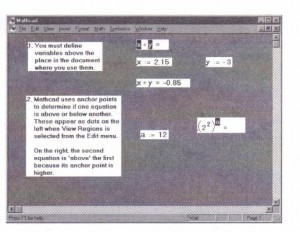# Recursive function definitions MathCad Help

Figure 7-4: The value of a user function depends on its arguments.
Pro Mathcad supports recursive function definitions-you may define the value of a function in terms of a previous value of the function. As shown in Figure 7-5, recursive functions are useful for defining arbitrary periodic functions, as well as elegantly implementing numerical functions like the factorial function. Note that a recursive function definition should always have at least two parts:
• An initial condition that prevents the recursion from going forever.
• A definition of the function in terms of some previous value(s) of the function. If you do not specify an initial condition that stops the recursion, Mathcad will generate a “stack overflow” error message as described in “Error messages” on page 148. The programming operators in Mathcad Professional also support

Mathoad scans a worksheet the same way you read it: left to right and top to bottom. This means that a variable or function definition involving a “: =” affects everything below and to the right of it. To determine whether one equation is above or below another, Mathcad compares their anchor points. To see these anchor points, choose Regions from the View menu. Mathcad will display blank space in gray and leave regions white (or whatever your background color happens to be). Each region’s anchor point will appear as a dot on the left.  Figure 7-6 shows an example of how not to place equations in a worksheet. In the first evaluation, both x and y are shown in red to indicate that they are undefmed. This is
because the definitions for x and y lie below where they are used. Because Mathcad scans from top to bottom, when it gets to the first equation, it has no idea what numbers to substitute in place of x and y. The second evaluation, on the other hand, is below the definitions of x and y. By the time Mathcad gets to this equation, it has already assigned values to both x and y.Figure 7-6: Mathcad evaluates equations from top to bottom in a worksheet.
The small dot on the left side of each equation is an anchor point. Undefined
variables are marked in reverse video.

You can define a variable twice in the same worksheet. Mathcad will simply use the first definition for all expressions below the first definition and above the second. For expressions below the second definition, Mathcad uses the second definition. Figure 7-7 illustrates a worksheet in which some variables are defined twice.

Global definitions

Globai definitions are exactly like local definitions except that they are evaluated before any local definitions. If you define a variable or function with a global definition, that variable or function is available to all local definitions in your worksheet, regardless of whether the local definition appears above or below the global definition. To type a global definition, follow these steps:
• Type a variable name or function to be defined.
• Press the tilde (-) key. The global definition symbol appears. 1 R
• Type an expression. The expression can I involve numbers or other globally defined 1R a .082056211 variables and functions.
You can use global definitions for functions, subscripted variables, and anything else that normally uses the definition symbol “:=”. Just type a tilde instead of a colon, and Mathcad will show the global definition symbol “=” in place of “:=” . This is the algorithm that Mathcad uses to evaluate all definitions, global and otherwise:
• First, Mathcad takes one pass through the entire worksheet from top to bottom. During this first pass, Mathcad evaluates global definitions only.Although global definitions are evaluated before any local definitions, Mathcad evaluates global definitions the same way it evaluates local definitions: top to bottom and left to right. This means that whenever you use a variable to the right of a “=”:
• that variable must also have been defined with a “=,” and
• the variable must have been defined above the place where you are trying to use it. Otherwise, the variable is marked in red to indicate that it is undefined, It is good practice to allow only one definition for each global variable. Although you can do things like define a variable with two different global definitions or with one global and one local definition, this is never necessary and usually serves only to make your worksheet difficult to understand.

Posted on November 21, 2015 in Equations and Computation